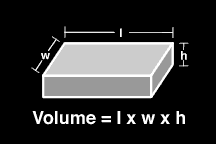Calculating Material Requirements
For A Project

Step One:

Calculate the volume of the part (or mold) in cubic inches. Follow whichever shape is closest to your model or mold:Step Two:

Find the volumetric yield for the material you have selected. This number, which can be found in most specification tables, represents how much coverage your material will achieve.

 If the Volumetric Yield is not available, you can calculate it based on the specific gravity (density). 1. Find the specific gravity (or density) of the material (on the specification table and/or MSDS). This is measured in grams per cubic centimeter (grams/cm3) 2. Take 27.68 divided by the density (grams/cm3) and this gives you the Volumetric Yield (in3/lb.)

Step Three:

Divide the volume of the part in cubic inches (step 1) by the volumetric yield (step 2) to determine the amount of material required in pounds.

 Volume of Model or Mold (in.3) --------------------------------------------- Volumetric Yield (in3/lb.) = Weight of Product Required (lbs.)

Step Four:

Select package size and quantity based on calculated weight of product required.

+